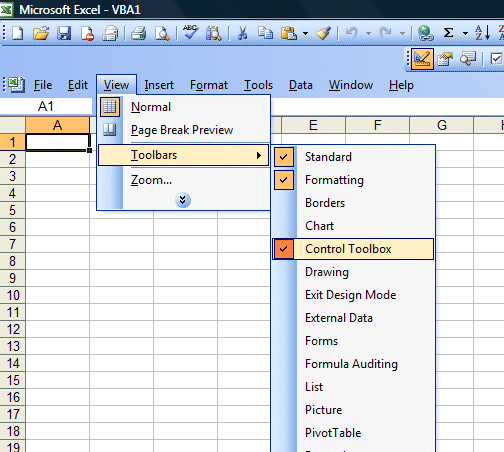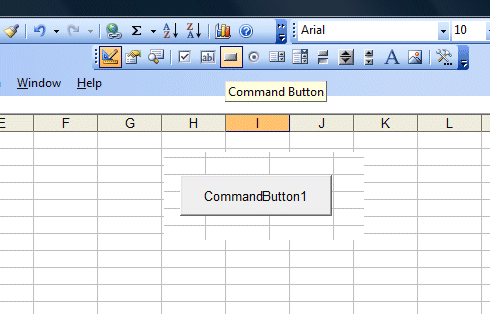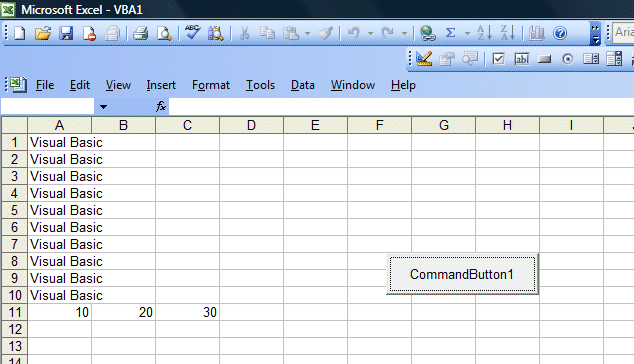# Excel VBA Lesson 1: Introduction to Excel VBA

### 1.1 What is Excel VBA?

Excel VBA is the acronym for Excel Visual Basic for Applications. It is an integration of the Microsoft’s event-driven programming language Visual Basic for Microsoft Office Applications such as Microsoft Excel. Using the Visual Basic programming language in Excel VBA, you can write customized solutions for the Microsoft Excel spreadsheet and complement its built-in functions. In addition, you can actually learn the fundamentals of Visual Basic programming without having to buy a copy of Visual Basic professional. Why is it so? It is because there is a built-in Visual Basic Editor in Microsoft Excel.

### 1.2  How to write Excel VBA Code?

There are two ways which you can write EXCEL VBA code. The first one is to place a command button on the spreadsheet and start writing codes by clicking the command button while the second one is to write Visual Basic codes within the Visual Basic Editor. Let’s start with the command button first. In order to place a command button on the spreadsheet, you need to click View on the MS Excel menu bar and then click on the toolbar and finally select the Control Toolbox to launch the control toolbox bar, as shown in Figure 1.1.Figure 1.1

The control toolbox comprises various controls. In this lesson, you only use the command button. Now click on the command button and draw it on the spreadsheet, as shown in Figure 1.2Figure 1.2

Next,  click on the command button to bring up the Visual Basic Editor. In the Visual Basic Editor,  enter the statements as shown in Figure 1.2. The first statement fills up cell A1 to cell A10 with the phrase “Visual Basic” while the second statement adds the value in cell A11 and cell B11  and then shows the sum in cell C11. It is that simple.Figure 1.2

Run the macro and you can see the output as shown in Figure 1.3  below:Figure 1.3